# 小学数学知识点例题讲解—几何中的计数问题

2020-03-25 13:10:03 浏览：

# 小学数学知识点例题讲解—几何中的计数问题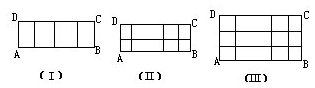4+3+2+1=10（个）.

（4+3+2+1）×（2+1）=10×3=30（个）.

4+3+2+1=10（个）.

（4+3+2+1）×（2+1）=10×3=30（个）.

（4+3+2+1）×（3+2+1）=10×6=60（个）.

（1+2+3+…+m）×（1+2+3+…+n）.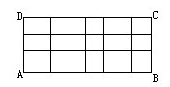BC边上分成的线段有：3+2+1=6.

（5+4+3+2+1）×（3+2+1）

=15×6

=90（个）.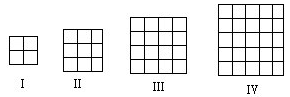1×1+2×2+3×3+4×4+5×5=55（个）.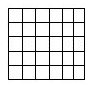①以一条基本线段为边的正方形个数共有：

6×5=30（个）.

②以二条基本线段为边的正方形个数共有：

5×4=20（个）.

③以三条基本线段为边的正方形个数共有：

4×3=12（个）.

④以四条基本线段为边的正方形个数共有：

3×2=6（个）.

⑤以五条基本线段为边的正方形个数共有：

2×1=2（个）.

6×5+5×4+4×3+3×2+2×1

=30+20+12+6+2=70（个）.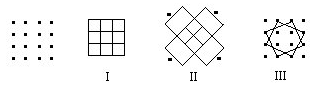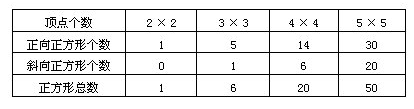Ⅰ.以一条基本线段为边的三角形：

①尖朝上的三角形共有四层，它们的总数为：

W①上=1+2+3+4=10（个）.

②尖朝下的三角形共有三层，它们的总数为：

W①下=1+2+3=6（个）.

Ⅱ.以两条基本线段为边的三角形：

①尖朝上的三角形共有三层，它们的总数为：

W②上=1+2+3=6（个）.

②尖朝下的三角形只有一个，记为W②下=1（个）.

Ⅲ.以三条基本线段为边的三角形：

①尖朝上的三角形共有二层，它们的总数为：

W③上=1+2=3（个）.

②尖朝下的三角形零个，记为W③下=0（个）.

Ⅳ.以四条基本线段为边的三角形，只有一个，记为：

W④上=1（个）.

Ⅰ.尖朝上的三角形共有四种：

W①下=1+2+3+4=10

W②上=1+2+3=6

W③上=1+2=3

W④上=1

Ⅱ.尖朝下的三角形共有二种：

W①下=1+2+3=6

W②下=1

W③下=0

W④下=0

20+7=27（个）.

Ⅰ.尖朝上的三角形有五种：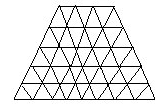（1）W①上=8+7+6+5+4=30

（2）W②上=7+6+5+4=22

（3）W③上=6+5+4=15

（4）W④上=5+4=9

（5）W⑤上=4

∴尖朝上的三角形共有：30+22+15+9+4=80（个）.

Ⅱ.尖朝下的三角形有四种：

（1）W①下=3+4+5+6+7=25

（2）W②下=2+3+4+5=14

（3）W③下=1+2+3=6

（4）W④下=1

80+46=126（个）